# Crack coding interviews by building these 5 real-world features

Mar 04, 2021 - 12 min read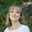Amanda FawcettPreparing for coding interviews is no easy task. You need the skills to break down the problem and to deploy the right tools. Educative has always been on the mission to make coding interview prep more accessible for engineers. We’ve learned firsthand that the best way to succeed is not to memorize 1500+ Leetcode problems.

That’s why we want to approach interview prep a bit differently today by tackling some real world problems faced by tech companies. Learning how to build real world features (like “how to merge recommendations on Amazon”) is more fun, and it’s much easier to remember what you learned. If you can understand a problem’s underlying pattern, you can apply it to just about any question.

We will dive into the solutions for a few common real world coding problems and build 5 features. We will offer our solutions in Java and Python.

This tutorial at a glance:

## Netflix Feature: Group Similar Titles (hash maps)

Netflix is one of the biggest video streaming platforms out there. The Netflix engineering team is always looking for new ways to display content. For this first problem, imagine you’re a developer on these teams.

Task: Our task here is to improve search results by enabling users to see relevant search results without being hindered by typos, which we are calling the “Group Similar Titles” feature.

First, we need to determine how to individually group any character combination for a given title. Let’s imagine that our library has the following titles: "duel", "dule", "speed", "spede", "deul", "cars". You are tasked to design a functionality so that if a user misspells a word (for example speed as spede), they will still be shown the correct title.

First, we need to split our titles into sets of words so that the words in a set are anagrams. We have three sets: {"duel", "dule", "deul"},{"speed", "spede"}, and {"cars"}. The search results should include all members of the set that the string is found in.

Note: It’s best to pre-compute our sets rather than forming them when a user searches.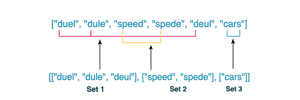Combining Similar Groups

The members of each set have the same frequency of each alphabet, so the frequency of each alphabet in words in the same group is equal. For example, in our set {{"speed", "spede"}}, the frequency of the characters are the same in each word: s, p, e, and d.

So, how do we design and implement this functionality? Let’s break it down.

1. For each title, we need to compute a 26-element vector. Each vector element represents the frequency of an English letter in a title. In Java, we can represent frequency using a string that is fixed with # characters. For Python, we can represent the count as a tuple. This mapping process generates identical vectors for the strings that are anagrams. So, for Java, we represent abbccc as #1#2#3#0#0#0...#0, and for Python, abbccc will be represented as (1, 2, 3, 0, 0, ..., 0).

2. We then use this vector as a key for inserting titles into a Hash Map. Our anagrams will all be mapped to the same entry. When a user searches a title or word, it should compute the 26-element English letter frequency vector based on input. It will then search the Hash Map and return all the map entries using the vector.

3. We then store a list of calculated character counts as a key in a Hash Map and assign a string as its value.

4. Each value is an individual set, so we return the values of the Hash Map.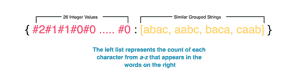Storing sets in a key-value storage with Java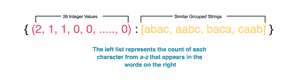Storing sets in a key-value storage with Python

### Practice and Solution

class Solution {
public static List<List<String>> groupTitles(String[] strs) {

return new ArrayList<List<String>>();
}
}
Try it yourself here before checking the solution
import java.util.*;
class Solution {
public static List<List<String>> groupTitles(String[] strs){
if (strs.length == 0)
return new ArrayList<List<String>>();

Map<String, List<String>> res = new HashMap<String, List<String>>();

int[] count = new int;
for (String s : strs) {
Arrays.fill(count, 0);
for (char c : s.toCharArray()){
int index = c - 'a';
count[index]++;
}

StringBuilder delimStr = new StringBuilder("");
for (int i = 0; i < 26; i++) {
delimStr.append('#');
delimStr.append(count[i]);
}

String key = delimStr.toString();
if (!res.containsKey(key))
res.put(key, new ArrayList<String>());

}

return new ArrayList<List<String>>(res.values());
}

public static void main(String[] args) {
// Driver code
String titles[] = {"duel","dule","speed","spede","deul","cars"};

List<List<String>> gt = groupTitles(titles);
String query = "spede";

// Searching for all titles
for (List<String> g : gt){
if (g.contains(query))
System.out.println(g);
}
}
}
Solution in Java and Python

### Complexity measures

$n$ is the size of the list of strings, and $k$ is the maximum length of a single string.

Time Complexity: $O(n*k)$, since we are counting each letter for each string in a list

Space Complexity: $O(n*k)$, since every string is stored as a value in the dictionary, and the size of the string can be $k$.

## Facebook Feature: Friend Circles (DFS)

Facebook is the biggest social media company in the world. They also own and operate Instagram. Pretend you are a Facebook engineer team, and you are tasked to improve integration among their sister platforms.

Task: Our task here is to find all the people on Facebook that are in a user’s friend circle, which we are calling the “Friend Circles” feature.

We need to first identify the people that are in each user’s friends circle, which includes users that are directly or indirectly friends with another user. Let’s assume there are $n$ users on Facebook. The friendship connection is transitive.

Example: If Nick is a direct friend of Amy, and Amy is a direct friend of Matt, then Nick is an indirect friend of Matt.

We will use an $n*n$ square matrix. For example, cell [i,j] will hold value 1 if these users are friends. If not, the cell will hold the value 0. In the illustration below, there are two friend circles in the above example. Nick is only friends with Amy, but Amy is friends with Nick and Matt. This forms a friend circle. Mario makes another friend circle on his own.

Think of our symmetric input matrix as an undirected graph. Both the indirect and direct friends who are in one friend circle also exist in one connected component​ in our graph. This means that the number of connected graph components will give us how many friend circles we have.

So, our task is to find the number of connected components. We treat the input matrix as an adjacency matrix. So, how do we design and implement this? Let’s break it down.

1. First we initialize an array, named visited. This will track the visited vertices of size n with 0 as the value for each index.
2. Then, we traverse the graph using DFS if visited[v] is 0. If not, we move to the next v.
3. Then, set visited[v] to 1 for every v that our DFS traversal runs into.
4. When the DFS traversal is done, we should increment the circles counter by 1. This means that a connected component has been fully traversed.

### Practice and Solution

class Solution {
public static void DFS (boolean[][] friends, int n, boolean[] visited, int v) {
for (int i = 0; i < n; ++i) {

return 0;
}
}
Try it yourself here before checking the solution
class Solution {

public static void DFS (boolean[][] friends, int n, boolean[] visited, int v) {
for (int i = 0; i < n; ++i) {

// A user is in the friend circle if he/she is friends with the user represented by
// user index and if he/she is not already in a friend circle
if (friends[v][i] == true && !visited[i] && i != v) {
visited[i] = true;
DFS(friends, n, visited, i);
}
}
}

public static int friendCircles(boolean[][] friends, int n) {
if (n == 0) {
return 0;
}

int numCircles = 0;     //Number of friend circles

//Keep track of whether a user is already in a friend circle
boolean visited[] = new boolean[n];

for (int i=0;i < n; i++){
visited[i] = false;
}

//Start with the first user and recursively find all other users in his/her
//friend circle. Then, do the same thing for the next user that is not already
//in a friend circle. Repeat until all users are in a friend circle.
for (int i = 0; i < n; ++i) {
if (!visited[i]) {
visited[i] = true;
DFS(friends, n, visited, i); //Recursive step to find all friends
numCircles = numCircles + 1;
}
}

return numCircles;
}

public static void main(String args[])
{
int n = 4;
boolean[][] friends = {
{true,true,false,false},
{true,true,true,false},
{false,true,true,false},
{false,false,false,true}
};
System.out.println("Number of friends circles: " + friendCircles(friends, n));
}

}
Solution in Java and Python

### Complexity measures

Time Complexity: $O(n^2)$ because we traverse the complete matrix of size $n^2$

Space Complexity: $O(n)$ because the visited array that stores our visited users is of size $n$.

## Google Calendar Feature: Find Meeting Rooms (heaps)

The Google Calendar tool is part of the GSuite used to manage events and reminders. Imagine you are a developer on the Google Calendar application team, and you’re tasked with implementing some productivity enhancing features.

Task: Your goal is to create a functionality for scheduling meetings. You need to determine and block the minimum number of meeting rooms for these meetings.

To do this, we are given a some meeting times. We need to find a way to identify the number of meeting rooms needed to schedule them all. Each meeting will contain positive integers for a startTime and an endTime.

Our meeting times can be listed as follows: {{2, 8}, {3, 4}, {3, 9}, {5, 11}, {8, 20}, {11, 15}}. We could schedule each meeting in a separate room, but we want to use the minimum amount of rooms. We observe that three meetings overlap: {2, 8}, {3, 4}, and {3, 9}. Therefore, at least these three will require separate rooms.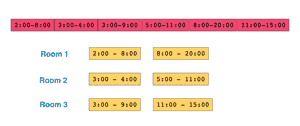To solve this, we use either a heap or priority queue for storing meeting timings, using end_time of each meeting as a key. The room at the top of our heap would become free earliest. If the meeting room from the top of the heap is not free, then no other room is free yet.

So, how do we build this functionality? Let’s break it down.

1. Sort the meetings by startTime.
2. Allocate the first meeting to a room. Add the endTime as an entry in the heap.
3. Traverse the other meetings and check if the meeting at the top has ended.
4. If the room is free, extract this element, add it to the heap again with the ending time of the current meeting we want to process. If it is not free, allocate a new room and add it to our heap.
5. After processing the lis of meetings, the size of the heap will tell us how many rooms are allocated. This should be the minimum number of rooms we need.

### Practice and Solution

import java.util.Arrays;
import java.util.PriorityQueue;
class Solution {

public static int minMeetingRooms(int[][] meetingTimes){

if(meetingTimes.length == 0){
return 0;
}
// write your code here 
Try it yourself here before checking the solution below
import java.util.Arrays;
import java.util.PriorityQueue;
class Solution {

public static int minMeetingRooms(int[][] meetingTimes){

if(meetingTimes.length == 0){
return 0;
}

Arrays.sort(meetingTimes, (a, b) -> Integer.compare(a, b));
//min Heap keeps track of earliest ending meeting:
PriorityQueue<Integer> minHeap = new PriorityQueue<>((A, B) -> A - B);

for (int i = 1; i < meetingTimes.length; i++) {
int currStart = meetingTimes[i];
int currEnding = meetingTimes[i];
int earliestEnding = minHeap.peek();

if (earliestEnding <= currStart) {
minHeap.remove();
}
//update heap with curr ending
}
return minHeap.size();
}

public static void main( String args[] ) {
// Driver code
int[][] meetingTimes = {{2, 8}, {3, 4}, {3, 9}, {5, 11}, {8, 20}, {11, 15}};
System.out.print(minMeetingRooms(meetingTimes));
}
}
Solution in Java and Python

### Complexity Measures

Time complexity: $O(n*log(n))$

Space complexity: $O(n)$, where $n$ is the number of meetings

### Reimagine your coding interview prep

Stop grinding through endless practice questions, and start breaking down 100+ real-world problems. Practice as you learn with hands-on coding environments inside your browser. No need to switch to your IDE, download an SDK, or scrub through videos.

Decode the Coding Interview: Real-World Examples

## Amazon Feature: Products in Price Range (BST)

Amazon is the largest online store around the world, and they are always on the lookout for better ways to recommend products to their customers. Imagine you are a developer for Amazon’s store.

Task: Implement a search filter to find products in a given price range. The product data is in the form of a binary search tree. The values are the prices of products.

The parameters we are working with are low and high, which represent a user’s price range. The example list of products below are mapped to their prices.They are then stored in a binary search tree:

We can assume that the selected price range is low = 7 and high = 20, so our function solution should only return the prices {9, 8, 14, 20, 17}. So, how do we implement this? Let’s break it down.

We will solve this problem using a variation of a preorder traversal on a binary tree, but other binary tree traversals would work as well.

1. Implement a recursive helper function for a preorder traversal.
2. Check heck if the current node value is in our given range. If so, add it to the output array.
3. Recursively call the preorder function on the left child of the node if the current node value greater than or equal to low.
4. If the current node value is less than or equal to high, recursively traverse the right child of the node.
5. When traversal is completed, the output array will be returned.

### Practice and Solution

main.java
BinarySearchTree.java
public class BinarySearchTree{
public Node root;
public BinarySearchTree(){
this.root = null;
}

// write your algorithm here
Try it yourself here before checking the solution
main.java
BinarySearchTree.java
public class BinarySearchTree{
public Node root;
public BinarySearchTree(){
this.root = null;
}

public void insert(int val){
if (this.root == null)
this.root = new Node(val);
else
this.root.insert(val);
}
}

class Node{
public int val;
public Node leftChild;
public Node rightChild;

public Node(int val){
this.val = val;
this.leftChild = null;
this.rightChild = null;
}

public void insert(int val){
Node current = this;
Node parent = current;
while (current != null){
parent = current;
if (val < current.val)
current = current.leftChild;
else
current = current.rightChild;
}
if(val < parent.val)
parent.leftChild = new Node(val);
else
parent.rightChild = new Node(val);
}
}
Solution in Java and Python

### Time complexity

Time complexity: $O(n)$

Space complexity: $O(n)$

Twitter is a popular social media platform. Imagine you’re a Twitter developer, and your team must create an API that calculates the number of likes on a given person’s Tweets.

Task: Create an API that calculates the total number of likes on a person’s Tweets. Create a module that takes two numbers and returns the sum of the numbers.

The data is already extracted and stored in a simple text file for you. All of the values should remain strings and cannot be converted into integers. We must do digit-by-digit addition due these restrictions. So, how do we create this module? Let’s break it down.

1. Initialize an empty res variable and the carry as 0.
2. Then, set two pointers, p1 and p2, that will point to the end of like1 and like2.
3. Traverse the strings from the end using these pointers. Set it to stop when both strings are done.
4. Set x1 equal to a digit from string like1 at index p1. If p1 has reached the beginning of like1, set x1 to 0. Do the same for x2 with like2 at index p2.
5. Compute the current value using value = (x1 + x2 + carry) % 10. Then update the carry so that carry = (x1 + x2 + carry) / 10.
6. Append the current value to the result.
7. If both of the strings are traversed but the carry is still non-zero, append the carry to res.
8. Lastly, reverse the result and convert it to a string. Return the final string.

### Practice and Solution

class Solution {
public static String addLikes(String like1, String like2){
StringBuilder res = new StringBuilder();

// write your code here
Try it yourself here before checking the solution
class Solution {
public static String addLikes(String like1, String like2){
StringBuilder res = new StringBuilder();

int carry = 0;
int p1 = like1.length() - 1;
int p2 = like2.length() - 1;
while (p1 >= 0 || p2 >= 0) {
int x1, x2;
if(p1 >= 0)
x1 = like1.charAt(p1) - '0';
else
x1 = 0;
if(p2 >= 0)
x2 = like2.charAt(p2) - '0';
else
x2 = 0;
int value = (x1 + x2 + carry) % 10;
carry = (x1 + x2 + carry) / 10;
res.append(value);
p1--;
p2--;
}

if (carry != 0)
res.append(carry);

return res.reverse().toString();
}
public static void main(String args[]) {
}
}
Solution in Java and Python

### Complexity measures

Time complexity: $O(max(n_1,n_2))$, where $n_1$ and $n_2$ are the lengths of the input.

Space complexity: $O(max(n_1,n_2))$ because the length of the result is $\max(N_1, N_2) + 1$

## Where to go from here

Congrats on making it to the end! You just build 5 real-world features, using skills like DFS, BST, heaps, and more. As you can see, this is a powerful way to prepare for coding interviews that tends to be more effective. If you can understand a problem’s underlying pattern, you can solve just about any question.

There are still dozens of other practice problems like this out there, like:

• Merge Tweets in Twitter Feed
• AT&T Determine Location
• Zoom: Display Lobby Meeting
• Search Engine: Fetch Words

If you want practice with problems like these, check out Educative’s one-of-a-kind course Decode the Coding Interview: Real-World Examples. Available in multiple languages, this course takes you through 100+ real-world questions like these. After each project, you’ll better understand what techniques you just applied.

This is coding interview prep reimagined, with your needs in mind!

Happy learning!